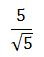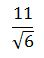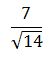# How to Rationalize the Denominator with a Radical Expression - Quiz & Worksheet

Instructions:

question 1 of 3

### Rationalize the denominator.Create Your Account To Take This Quiz

As a member, you'll also get unlimited access to over 84,000 lessons in math, English, science, history, and more. Plus, get practice tests, quizzes, and personalized coaching to help you succeed.

Try it risk-free for 30 days. Cancel anytime

### 1. What do you multiply by to rationalize this denominator?### 2. Rationalize the denominator.Create your account to access this entire worksheet
Quizzes, practice exams & worksheets
Certificate of Completion
Create an account to get started

Rationalizing denominators with radical expressions requires movement of this denominator to the numerator. This quiz and worksheet combo will help you test your understanding of this process. The quiz questions will test your understanding of how to perform this operation.

## Quiz & Worksheet Goals

In these assessments, you'll be tested on:

• Rationalizing denominators
• Identifying the steps needed to complete this process

## Skills Practiced

This quiz and worksheet will allow you to test your skills in the following areas:

• Reading comprehension - ensure that you draw the most important information about rationalizing radical denominators from the related lesson
• Critical thinking - apply relevant concepts to examine information about radical expressions in a different light
• Problem solving - use acquired knowledge to solve rationalization practice problems
• Information recall - access the knowledge you've gained regarding rationalizing radical denominators

To learn more about rationalizing denominators with radical expressions, review the accompanying lesson How to Rationalize the Denominator with a Radical Expression. This lesson covers the following objectives:

• Define irrational denominator as a radical denominator
• Understand how to rationalize a denominator by moving the radical to the numerator position
• Understand the process of moving the denominator to the numerator
Final ExamAlgebra II: High School
Status: Not Started
Chapter ExamAlgebra II: Real Numbers
Status: Not Started

Support